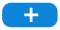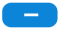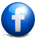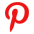### Wireless Telescopic Game Controller Compatible Phone8/xr/xs

Description:NOTE:NOT for iOSDescription:- This Gamepad supports playing games on Wireless enabled Android tablet/Smart Phone/TV box/Smart TV/ VR, Win 7/ Win 8/ Win 10 PC without any drivers.- Multimedia player buttons "volume +, -,"last ","next ""Play / Pause" can be used under Android system devices.- Support most gamepad enabled games downloaded from Google Play Store- Fit for 5-10 inch of mobile phones or tablets. Max. extending size is 11 inch (280mm)- Ergonomic design fits perfectly in your hands and feel great for hours of use- Support playing games on tablet in vertical or horizontal screen- High sensibility & accuracy button. Press test 1000000+NOTE:This is updated version of PG-9083, which is NOT compatible with Nintendo Switch.Specification:Working Voltage: DC 3.7VWorking Current: 30 mAConsecutive Usage Hours: 10hrsSleep Mode Current: 30uacharging voltage="" current:="" dc="" 5v="" 300mabattery="" capacity:="" 380mahwireless="" transmission="" range:="" 8m="" standby="" time:="" up="" to="" 30="" days="" once="" fully="" chargedpackage="" includes:1="" x="" pg-9083s="" wireless="" controller1="" user="" manual1="" micro="" usb="" charge="" cable2="" black="" plastic="" blocksabout="" this="" item1="" compatibility:="" not="" compatible="" with="" apple="" ios="" devices="" android="" 6="" 0="" and="" higher="" samsung="" galaxy="" s9="" s10="" note="" 10="" s20="" 5g="" huawei="" mate="" 20="" 20plus="" p30="" p40="" pro="" m40="" oppo="" vivo="" x27="" x30="" xiaomi="" lg="" smartphones="" tablets="" note:="" please="" make="" sure="" the="" system="" is="" or="" before="" purchasing="" phone="" does="" support="" mediatek="" chip="" phones="" 2="" startup="" method:="" customers="" need="" select="" pairing="" method="" according="" device="" there="" a="" mode="" button="" on="" left="" side="" of="" handle="" are="" two="" modes:="" v3="" direct="" standard="" game="" after="" selecting="" correctly="" press="" hold="" home="" start="" led="" indicator="" flashes="" quickly="" turn="" pair="" connection="" successful="" will="" always="" be="" 3="" download="" shootingplus="" app:="" use="" gamepad="" for="" first="" time="" customer="" needs="" customize="" settings="" your="" scan="" qr="" code="" manual="" follow="" app="" buttons="" customizations="" their="" own="" habits="" set="" 4="" when="" setting="" you="" should="" changes="" adjust="" size="" virtual="" joystick="" appropriately="" during="" avoid="" too="" many="" blind="" spots="" align="" them="" inoperability="" can="" search="" related="" video="" tutorials="" pg-9083s-shootingplus-v3="" youtube="" if="" have="" any="" questions="" contact="" us="" in="" send="" an="" email="" 5="" adopts="" solution="" which="" most="" games="" market="" structure="" accords="" ergonomics="" continuous="" hitting="" function="" turbine="" makes="" more="" exciting="" comfortable="" operate="" accurate="" positioning="" stretching="" 280mm="" 11="" inches="" built-in="" 380mah="" high-performance="" lithium="" battery="" used="" continuously="" than="" 15="" hours="" charged="" span="">

# Wireless Telescopic Game Controller Compatible Phone8/xr/xs

\$49.99
(including GST)Like on Facebook, click it:Share this deal on Facebook: Share nowTwitt on Twitter, click it:Share this deal on Pinterest: